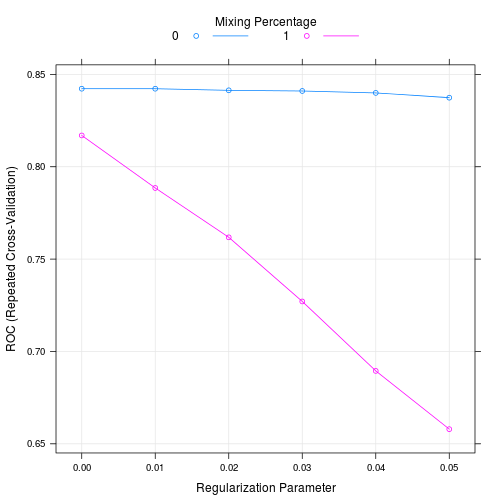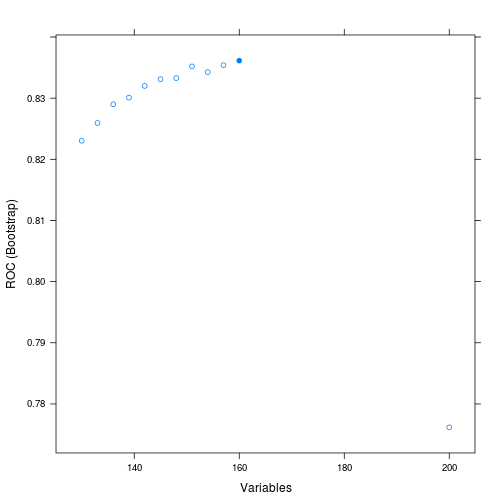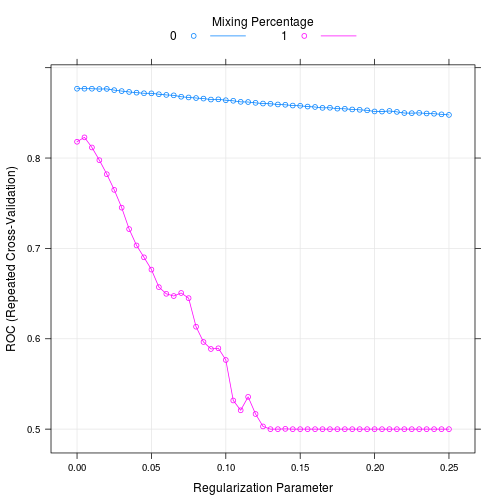# Don't Overfit

The purpose of this competition is too predict the values of a 19,750 element test set based on a 250 element training set. Each set has 200 variables to classify each element. The purpose of this project is to fit the data using Random Forests. As the training set has very few elements, it will be neccessary to reuse elements in a boot strap method, but not so much as to overfit the data.

Random Forests are computationally expensive, so it is helpful to parrellize the process, and run it on multiple cores. This speeds up computation time, allowing for more robust methods to be used to analyze data. This project is done on a Lonovo Y500, running Linux Ubuntu 13.1. Windows users will have to modify the multicore step for the program to properly run.

First install and load the required packages and dependencies. Caret is for building predictive models, reshape2 and plyr are for data management, and caTools is to score the models using a Receiver Operating Characteristic (ROC) curve. In this step, the working directory is also set for the duration of the program.

``````install.packages(c("caret","reshape2","plyr","caTools"),dependencies=c("Depends", "Imports", "LinkingTo", "Suggests", "Enhances"))
setwd("C:/Users/Jamie/Dropbox/Group8/Long assignment/3. Jamie - Kaggle Competition Walkthrough")
``````

Next load the packages reqired for analysis. I have also used set.seed to specify a starting value for random numbers so that my results can be replicated exactly. Random Forests have a stochastic element, and as a result, will produce similar, but not identical results every time the program is run unless set.seed is specified. Computers simulate random numbers based on previouse random numbers, so pseudo-random is a better term. The set.seed function specifies the first random number to use, and as a result, all of the random numbers that the function will use if left unchanged.

A Random Forest selects a subset of data at random from the training data, and a subset of variables ar random from all of the variables in the the training set. The selection process in completed randomly. The method then fits a tree with the subset of variables, to the subset of data as closely as possible. Repeat many times. The collection of trees is the forest, and will be averaged to predict test values, and performance will be evaluated based on this cross validation.

``````set.seed(250)
options(warn = -1)

library("caret")
``````
``````## Loading required package: lattice
## Loading required package: ggplot2
``````
``````library("glmnet")
``````
``````## Loading required package: Matrix
## Loaded glmnet 1.9-5
``````
``````library("ipred")
``````
``````## KernSmooth 2.23 loaded
## Copyright M. P. Wand 1997-2009
``````
``````library("e1071")
``````
``````## Loading required package: class
``````
``````library("caTools")
``````

The next step is to import, and organize the data. Import using the read.csv function. The file is not too large, so this step takes very little time to execute ~2 seconds. I then convert the 1s and 0s in the TargetPractice column to X1 and X0 so that R recognizes this is a classification problem instead of a regression problem, and assign this to a new column called Target. The values in TargetEvaluate, TargetLeaderboard, and TargetPractice can then be removed as they will only be needed once the model has been trained, and it is easier to call them later than to keep them in memory, and risk training a model to them.

Organize the data so that Target is in the first column for reading into training functions. The functions that will be used require the dependent variable to be in the first column. At this point, Target_Practice is the only column of importance. Tune and test the model with this column, and then fit it to the evaluation columns.

``````Data <- read.csv("overfitting.csv", header = TRUE)

# Choose Target
Data\$Target <- as.factor(ifelse(Data\$Target_Practice == 1, "X1", "X0"))
Data\$Target_Evaluate = NULL
Data\$Target_Leaderboard = NULL
Data\$Target_Practice = NULL

# Order
xnames <- setdiff(names(Data), c("Target", "case_id", "train"))
Data <- Data[, c("Target", "case_id", "train", xnames)]
``````

Data now needs to be separated into training and testing values. Luckily, these are already sorted in the data set, so only need to be organized. Remove this column once sorted. Also, define FL as a function for later. This function uses xnames which is defined above,and is the names of the different variables in the dataset.

``````# Split to train and test
trainset = Data[Data\$train == 1, ]
testset = Data[Data\$train == 0, ]

# Remove unwanted columns
trainset\$case_id = NULL
trainset\$train = NULL

testset\$case_id = NULL
testset\$train = NULL

# Define Formula
FL <- as.formula(paste("Target ~ ", paste(xnames, collapse = "+")))
``````

The data set is now organized to perform analysis. Use the head() and tail() functions to view the data, but it is too large, and not neccessary to present in this example.

The next steps involve fitting models, which for random forests is computationally expensive. To speed up comutation time, spread the work out over more computer cores. The code for this step will vary by machine and operating system. The code below is designed for windows 8.1, and is run on a lenovo Y500 machine.

R is designed to only run on one core, but many computers have at least 2. By assigning the process of fitting the trees to more cores, computation time is significantly decreased. The below code will split the fitting process into mini processes, and each mini process to a seperate core. The individual cores will complete the process, and send it back into the master system, thus speeding up the process. Running something as computationally expensive as fitting thousands of trees on one core is analagous to driving a Ferrari in first gear.

``````library("multicore")
``````
``````##
## Attaching package: 'multicore'
##
## The following object is masked from 'package:lattice':
##
##     parallel
``````

Define the MyTrainControl parameter, which will be assigned to the train function of caret. This specifies the number of cross validation sets to use, and the number of times to repeat it. As this competition is judged on probabilities, the output is required to be in a probabalistic form. The twoClassSummary specifies the metrics to ouput for evaluation purposes.

``````MyTrainControl = trainControl(method = "repeatedCV", number = 10, repeats = 5,
returnResamp = "all", classProbs = TRUE, summaryFunction = twoClassSummary)
``````

Apply this MyTrainControl paramerter to train the model. A demonstration of the model, and the parameters is shown below:

``````model <- train(FL, data = trainset, method = "glmnet", metric = "ROC", tuneGrid = expand.grid(.alpha = c(0,
1), .lambda = seq(0, 0.05, by = 0.01)), trControl = MyTrainControl)
``````
``````## Loading required package: pROC
## Type 'citation("pROC")' for a citation.
##
## Attaching package: 'pROC'
##
## The following object is masked from 'package:glmnet':
##
##     auc
##
## The following object is masked from 'package:stats':
##
##     cov, smooth, var
``````
``````model
``````
``````## glmnet
##
## 250 samples
## 200 predictors
##   2 classes: 'X0', 'X1'
##
## No pre-processing
## Resampling: Cross-Validated (10 fold, repeated 5 times)
##
## Summary of sample sizes: 225, 225, 225, 225, 225, 225, ...
##
## Resampling results across tuning parameters:
##
##   alpha  lambda  ROC  Sens  Spec  ROC SD  Sens SD  Spec SD
##   0      0       0.8  0.8   0.8   0.08    0.1      0.09
##   0      0.01    0.8  0.8   0.8   0.08    0.1      0.09
##   0      0.02    0.8  0.7   0.8   0.08    0.1      0.09
##   0      0.03    0.8  0.7   0.8   0.08    0.1      0.1
##   0      0.04    0.8  0.7   0.8   0.08    0.1      0.1
##   0      0.05    0.8  0.8   0.8   0.08    0.1      0.1
##   1      0       0.8  0.7   0.7   0.08    0.1      0.1
##   1      0.01    0.8  0.6   0.8   0.09    0.2      0.1
##   1      0.02    0.8  0.7   0.7   0.1     0.2      0.2
##   1      0.03    0.7  0.6   0.7   0.1     0.2      0.1
##   1      0.04    0.7  0.5   0.7   0.1     0.2      0.2
##   1      0.05    0.7  0.5   0.7   0.1     0.2      0.1
##
## ROC was used to select the optimal model using  the largest value.
## The final values used for the model were alpha = 0 and lambda = 0.01.
``````
``````plot(model, metric = "ROC")
``````It is evident that xxx. Now, a demonstration of how well the best performing model predicts the test set.

``````test <- predict(model, newdata = testset, type = "prob")
colAUC(test, testset\$Target)
``````
``````##               X0     X1
## X0 vs. X1 0.8679 0.8679
``````

The result is ~87% accuracy which is just a little below the the benchmark, but is in the same ballpark. A good first try. Now searh for imporvements to beat the benchmark.Create a custom function to do this.

Assign the values of the default caret functions to a new variable, that will also take twoClassSummary explained above. The new function will rank and sort variables to use in the final model. It is important to not fit the model to too many variables and risk overfitting, so select only the most important variables in predicting the outcome. The function essentially fits a model, ranks the parameters, and removes the least important ones in an iterative fashion.

The rfe function in the caret package has a the ability to select the best variables to use in the final model, as determined by cross validation. The function samples the data, determines variable importance, and removes the least important variable. The process iterates through this process, while keeping track of the results at each step. The parameters of the best fitting model are then returned. The rank function determines rank by coefficients of the variables in the function, where a higher coefficient corresponds to a higher rank. The number parameter specifies the number of repeats of bootstrapping to do in each loop. A higher number is more accurate, but takes longer to run.

``````#################################### RFE parameters

# Custom Functions
glmnetFuncs <- caretFuncs  #Default caret functions

glmnetFuncs\$summary <- twoClassSummary

glmnetFuncs\$rank <- function(object, x, y) {
vimp <- sort(object\$finalModel\$beta[, 1])
vimp <- as.data.frame(vimp)
vimp\$var <- row.names(vimp)
vimp\$Overall <- seq(nrow(vimp), 1)
vimp
}

MyRFEcontrol <- rfeControl(functions = glmnetFuncs, method = "boot", number = 50,
rerank = FALSE, returnResamp = "final", saveDetails = FALSE, verbose = F)
``````

The next step is to set up the training and multicore parameters. Multicore is explained in detail above, and MyTrainControl if very similar to MyRFEControl.

RFE below tests the different fitted trees with varying numbers of parameters, always using the most important parameters. From the forum of the competition, it is already known that 145 is the optimal number of variables, so test from 130 - 160 returning a value for every third number to validate this conclusion. Alpha and lambda are tuning parameters, and are used to adjust the model to improve performance.

The workers, computeFunction, and computeArgs elements of MyRFEcontrol and MyTrainControl are being defined for the multicore package. This allows for the program to utilize the full capability of the machine for computation. Rather than running the program on 1 core, multicore divides the process among as many cores that exist, speeding up the computation time.

``````MyTrainControl = trainControl(method = "repeatedCV", number = 10, repeats = 50,
returnResamp = "all", classProbs = TRUE, summaryFunction = twoClassSummary)

MyRFEcontrol\$workers <- multicore:::detectCores()
MyRFEcontrol\$computeFunction <- mclapply
MyRFEcontrol\$computeArgs <- list(mc.preschedule = F, mc.set.seed = F)

MyTrainControl\$workers <- multicore:::detectCores()
MyTrainControl\$computeFunction <- mclapply
MyTrainControl\$computeArgs <- list(mc.preschedul = F, mc.set.seed = F)

x <- trainset[, xnames]
y <- trainset\$Target

RFE <- rfe(x, y, sizes = seq(130, 160, by = 3), metric = "ROC", maximize = TRUE,
rfeControl = MyRFEcontrol, method = "glmnet", tuneGrid = expand.grid(.alpha = 0,
.lambda = c(0.01, 0.02)), trControl = MyTrainControl)

NewVars <- RFE\$optVariables
plot(RFE)
````````````
FL <- as.formula(paste("Target ~ ", paste(NewVars, collapse = "+")))  #RFE
``````

Lastly, fit a glmnet model to the data. An RFE model was fit previously, but fitting a glmnet model allows for the alpha and lambda parameters to be varied, creating a better fit in the end. The model is used to predict the values in the test case below. The model is fairly accurate prediciting the correct value ~xxx% of the time, though not as accurate as the winner who was ~97% correct. However, the random forest method will be used in my final project, and can be built off of this code.

``````model <- train(FL, data = trainset, method = "glmnet", metric = "ROC", tuneGrid = expand.grid(.alpha = c(0,
1), .lambda = seq(0, 0.25, by = 0.005)), trControl = MyTrainControl)
plot(model, metric = "ROC")
````````````test <- predict(model, newdata = testset, type = "prob")
colAUC(test, testset\$Target)
``````
``````##              X0    X1
## X0 vs. X1 0.899 0.899
``````
``````
predictions <- test

testID <- testset\$case_id
submit_file = data.frame(Jamie = predictions[, 1])
``````